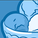cancel
Showing results for
Did you mean:Contributor III

## Taking the Sum of an already summed expression

Hi,

I am trying to take the sum of two expressions being multipled against each other, but one of the expressions is a rangesum, not allowing me to do so. Please see the attached screenshot for an example look of what I am trying to do. I am also trying to avoid doing the rangesum in the load script.

I want to multiply the below two expressions against each other, then after, take the sum of it.

Expression 1: Calculates the rolling 4 wk sum of my original expression: Sum(Qty)

=Avg(Aggr(RangeSum(Above(Sum({< YYYYQ =, YYYYWK = >} Qty)

, 0, 4)), Group, YYYYWK))

Expression 2: Is just the Amt.

=Sum(Amt)

So, what I need to do is:

SUM(

Avg(Aggr(RangeSum(Above(Sum({< YYYYQ =, YYYYWK = >} Qty)

, 0, 4)), Group, YYYYWK))

*

Amt

)

I think that because in my first expression, I am already taking the rangesum of my original expression, I cannot take the sum of the whole formula again.

Note: {< YYYYQ =, YYYYWK = >} is there just to say that upon making selections on these fields, do not change values. Can be disregarded on functionality, just needs to be included in syntax.

Any help would be greatly appreciated!

Regards

Labels (5)

• ### sum

1 Solution

Accepted SolutionsHi,

If you ever find yourself wanting to do one aggregation inside another you need an AGGR. This effectively builds a temporary table where you can get the results of the inner aggregation at every dimension defined by the AGGR parameters.

In your case you will have an AGGR within your AGGR, which may cause weirdness, but give this a try:

SUM(
aggr(
Avg(Aggr(RangeSum(Above(Sum({< YYYYQ =, YYYYWK = >} Qty)
, 0, 4)), Group, YYYYWK))
*
Amt,
Group,Person,YYYYWK)
)

Note that the fields listed as AGGR parameters for the outer parameters are taken from your screengrab, as these define the granularity you want the inner expression done to.

I think what you want to do can be simplified, as you do not want to see the table by week, and the rolling total can just be rolled up, effectively done on two rows. The code would then be:

sum(aggr(avg(Qty*Amt), Group, Person))

I may be missing something about what you are looking to achieve though?

Hope that makes sense.

Steve
3 RepliesHi,

If you ever find yourself wanting to do one aggregation inside another you need an AGGR. This effectively builds a temporary table where you can get the results of the inner aggregation at every dimension defined by the AGGR parameters.

In your case you will have an AGGR within your AGGR, which may cause weirdness, but give this a try:

SUM(
aggr(
Avg(Aggr(RangeSum(Above(Sum({< YYYYQ =, YYYYWK = >} Qty)
, 0, 4)), Group, YYYYWK))
*
Amt,
Group,Person,YYYYWK)
)

Note that the fields listed as AGGR parameters for the outer parameters are taken from your screengrab, as these define the granularity you want the inner expression done to.

I think what you want to do can be simplified, as you do not want to see the table by week, and the rolling total can just be rolled up, effectively done on two rows. The code would then be:

sum(aggr(avg(Qty*Amt), Group, Person))

I may be missing something about what you are looking to achieve though?

Hope that makes sense.

SteveContributor III
Author

Hi Steve,

This expression works perfectly:

SUM(
aggr(
Avg(Aggr(RangeSum(Above(Sum({< YYYYQ =, YYYYWK = >} Qty)
, 0, 4)), Group, YYYYWK))
*
Amt,
Group,Person,YYYYWK)
)

Thank you so much for the help on this, it really helped me out.